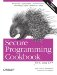# Getting the Length of a String

Problem

You need the length of a string.

Solution

Use string's length member function:

```std::string s = "Raising Arizona";
int i = s.length( );```

Discussion

Retrieving the length of a string is a trivial task, but it is a good opportunity to discuss the allocation scheme for strings (both wide and narrow character). strings, unlike C-style null-terminated character arrays, are dynamically sized, and grow as needed. Most standard library implementations start with an arbitrary (low) capacity, and grow by doubling the capacity each time it is reached. Knowing how to analyze this growth, if not the exact algorithm, is helpful in diagnosing string performance problems.

The characters in a basic_string are stored in a buffer that is a contiguous chunk of memory with a static size. The buffer a string uses is an arbitrary size initially, and as characters are added to the string, the buffer fills up until its capacity is reached. When this happens, the buffer grows, sort of. Specifically, a new buffer is allocated with a larger size, the characters are copied from the old buffer to the new buffer, and the old buffer is deleted.

You can find out the size of the buffer (not the number of characters it contains, but its maximum size) with the capacity member function. If you want to manually set the capacity to avoid needless buffer copies, use the reserve member function and pass it a numeric argument that indicates the desired buffer size. There is a maximum size on the possible buffer size though, and you can get that by calling max_size. You can use all of these to observe memory growth in your standard library implementation. Take a look at Example 4-9 to see how.

Example 4-9. String length and capacity

```#include
#include

using namespace std;

int main( ) {

string s = "";
string sr = "";

sr.reserve(9000);

cout << "s.length = " << s.length( ) << '
';
cout << "s.capacity = " << s.capacity( ) << '
';
cout << "s.max_size = " << s.max_size( ) << '
';

cout << "sr.length = " << sr.length( ) << '
';
cout << "sr.capacity = " << sr.capacity( ) << '
';
cout << "sr.max_size = " << sr.max_size( ) << '
';

for (int i = 0; i < 10000; ++i) {

if (s.length( ) == s.capacity( )) {
cout << "s reached capacity of " << s.length( )
<< ", growing...
";
}
if (sr.length( ) == sr.capacity( )) {
cout << "sr reached capacity of " << sr.length( )
<< ", growing...
";
}
s += 'x';
sr += 'x';
}
}```

With Visual C++ 7.1, my output looks like this:

```s.length = 0
s.capacity = 15
s.max_size = 4294967294
sr.length = 0
sr.capacity = 9007
sr.max_size = 4294967294
s reached capacity of 15, growing...
s reached capacity of 31, growing...
s reached capacity of 47, growing...
s reached capacity of 70, growing...
s reached capacity of 105, growing...
s reached capacity of 157, growing...
s reached capacity of 235, growing...
s reached capacity of 352, growing...
s reached capacity of 528, growing...
s reached capacity of 792, growing...
s reached capacity of 1188, growing...
s reached capacity of 1782, growing...
s reached capacity of 2673, growing...
s reached capacity of 4009, growing...
s reached capacity of 6013, growing...
sr reached capacity of 9007, growing...
s reached capacity of 9019, growing...```

What is happening here is that the buffer for the string keeps filling up as I append characters to it. If the buffer is full (i.e., length = capacity), a new, larger buffer is allocated and the original string characters and the newly appended character(s) are copied into the new buffer. s starts with the default capacity of 15 (results vary by compiler), then grows by about half each time.

If you anticipate significant growth in your string, or you have a large number of strings that will need to grow at least modestly, use reserve to minimize the amount of buffer reallocation that goes on. It's also a good idea to experiment with your standard library implementation to see how it handles string growth.

Incidentally, when you want to know if a string is empty, don't check length against zero, just call the empty member function. It is a const member function that returns true if the length of the string is zero.Secure Programming Cookbook for C and C++: Recipes for Cryptography, Authentication, Input Validation & More
ISBN: 0596003943
EAN: 2147483647
Year: 2006
Pages: 241